The evaluation of expressions can be affected by definitions assigned to  symbols
\$PreRead, MakeExpression, \$Pre, \$Post, \$PrePrint, Format,  MakeBoxes. The process of going from input cell to output cell is as follows.   Each of these steps requires evaluation of an expression, and that evaluation process is explained in a later section.

(1)  Input Boxes are processed  using \$PreRead.
(2)  The boxes \$PreRead returns are converted to an  expression using MakeExpression.
(3)  The expression MakeExpression returns is processed using \$Pre.
(4)  The expression \$Pre returns is evaluated.
(5)  The expression  returned by evaluation is processed by \$Post.
(6)  The expression \$Post  returns is assigned to (%n) and stored in DownValues[Out].
(7)  The  expression that was assigned to (%n) is processed by \$PrePrint.
(8)  The  expression \$PrePrint returns is processed by definitions made using Format.
(9)  The expression Format returns is converted to boxes using MakeBoxes.

Consider the input in the next cell.  The 2-D representation of the cell  is stored as
SqrtBox[
RowBox[{
FractionBox["35",  "100"], "+", "1"}]]

Before Mathematica can evaluate this input it converts it to the expression
Power[Plus[Times[35,Power[100,-1]],1],Rational[1,2]]

However, before  the boxes are converted to this expression Mathematica checks to see if the boxes should be changed using \$PreRead.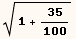The next cell cause \$Pre to change the box representation of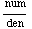into the box representation of MyDivide[num,den].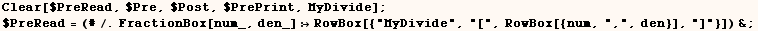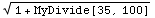The next cell removes the above \$PreRead assignment.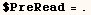\$Pre

In the next cell \$Pre is given the input before evaluation and changed any
occurance of 100 into the symbol Hundred.  Use of Unevaluated and HoldAll is
needed to ensure the expression doesn't evaluate before making the
replacement.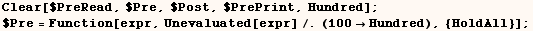Below we see that \$Pre makes the replacement before making the calulation
(35/100)→(7/20).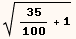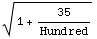The next cell removes the above \$Pre assignment.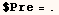\$Post

In the next cell we make \$Post display matrices in MatrixForm and all other
results are not affected. The symbols returned by evaluating \$OutputForms get
special treatment in that they affect the printed output, but not the results
assigned to (%n). By default \$OutputForms includes MatrixForm, TableForm,
ScientificForm and other built-in formatting functions.  Provided MatrixForm
is in the list returned by \$OutputForms we could have assigned the definition
below to \$PrePrint and got the same results.After making the assignment above the output of the following is
automatically displayed in MatrixForm.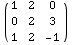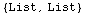The next cell makes it so MatrixForm isn't included in the \$OutputForms
listWhen MatrixForm isn't in the \$OutputForms list Head[%] below is MatrixForm.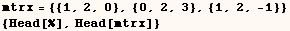The next cell restores the default setting of \$OutputForms and removes the
above \$Post setting.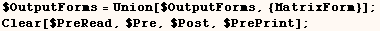\$PrePrint

\$Post and \$PrePrint are very similar and are normally used to affect the way
output is displayed.  For example in the next cell we make \$PrePrint display
matrices in MatrixForm and all other results are not affected. \$PrePrint is
used after results are assigned to %n, so use of \$PrePrint will not affect
assignments to (%n) regardless of what is assigned to \$OutputForms.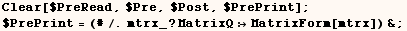After making the assignment above the output of the following is
automatically displayed in MatrixForm. Because of the way \$PrePrint works we
get List for Head[%] below regardless of what is assigned to \$OutputForms.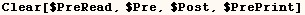\$Post versus  \$PrePrint  (a closer look)

Consider the use of \$PrePrint in the next cell.After making the previous assignment to \$PrePrint, the next output shows (1+
π) instead of simply π.  There is clearly no pratical use for this
sort of thing.  I am only doing this to illustrate the difference between
\$Post and \$PrePrint.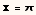The result stored with (%n) from the evaluation above is π because
\$PrePrint is used after the result is assigned to %n. This is demonstrated in
the next cell where the previous output is accessed using (%%).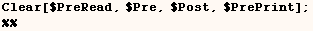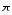In the next cell we use \$Post to do the same thing we did with \$PrePrint in
the previous example.  When we use \$Post the replacement is made before the
result is assigned to (%n).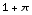In the next cell we see (1+π) is assigned to (%n) when the replacement is